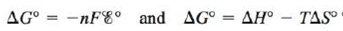# Combine the equations to derive an expression for as a function of temperature. Describe how one can graphically determine ∆ H ° and ∆ S ° from measurements of at different temperatures, assuming that ∆ H ° and ∆ S ° do not depend on temperature. What property would you look for in designing a reference half-cell that would produce a potential relatively stable with respect to temperature?### Chemistry: An Atoms First Approach

2nd Edition
Steven S. Zumdahl + 1 other
Publisher: Cengage Learning
ISBN: 9781305079243

#### Solutions

Chapter
Section### Chemistry: An Atoms First Approach

2nd Edition
Steven S. Zumdahl + 1 other
Publisher: Cengage Learning
ISBN: 9781305079243
Chapter 17, Problem 141CP
Textbook Problem
5 views

## Combine the equationsto derive an expression foras a function of temperature. Describe how one can graphically determine ∆H° and ∆S° from measurements ofat different temperatures, assuming that ∆H° and ∆S° do not depend on temperature. What property would you look for in designing a reference half-cell that would produce a potential relatively stable with respect to temperature?

Interpretation Introduction

Interpretation:

Two different equations showing the change in Gibbs free energy are given. The derivation of E° as a function of temperature for the given equations, the graphical determination of ΔH° and ΔS° from measurements of E° at different temperature and the property used for designing a reference half-cell that would produce a potential relatively stable with respect to temperature is to be stated.

Concept introduction:

Gibbs free energy is basically the maximum amount of non-expansion work done. Therefore, it is represented as,

Wmax=ΔG°

The relationship between Gibbs free energy change and cell potential is given by the formula,

ΔG°=nFE°cell

The relation between ΔG° , ΔH° and ΔS° is given as,

ΔG°=ΔH°TΔS°

To determine: The derivation of E° as a function of temperature for the given equations, the graphical determination of ΔH° and ΔS° from measurements of E° at different temperatures and the property used for designing a reference half-cell that would produce a potential relatively stable with respect to temperature.

The relation obtained from the given equations is,

E°cell=T(ΔS°nF)+(ΔH°nF)

### Explanation of Solution

The relationship between ΔG° , ΔH° and ΔS° is given as,

ΔG°=ΔH°TΔS° (1)

Where,

• ΔG° is the standard Gibbs free energy change.
• ΔH° is the standard enthalpy change.
• ΔS° is the standard entropy change.
• T is the temperature at which reaction occurs.

The relationship between Gibbs free energy change and cell potential is given by the formula,

ΔG°=nFE°cell (2)

Where,

• ΔG is the Gibbs free energy change at the given temperature conditions.
• n is the number of moles of electrons that are involved in the reaction.
• F is the Faraday’s constant (96,485C/molee) .
• Ecell is the cell potential at the given temperature conditions.

Combine equation (1) and (2) as,

nFE°cell=ΔH°TΔS°

Rearrange the equation to obtain the value of E°cell as,

E°cell=T(ΔS°nF)+(ΔH°nF) (3)

When the graph between E°cell and T is plotted, it leads to a straight line and the value of ΔH° and ΔS° is obtained from the obtained straight line equation.

The relationship between ΔG° , ΔH° and ΔS° is given as,

ΔG°=ΔH°TΔS° (1)

Where,

• ΔG° is the standard Gibbs free energy change.
• ΔH° is the standard enthalpy change.
• ΔS° is the standard entropy change.
• T is the temperature at which reaction occurs.

The relationship between Gibbs free energy change and cell potential is given by the formula,

ΔG°=nFE°cell (2)

Where,

• ΔG is the Gibbs free energy change at the given temperature conditions

### Still sussing out bartleby?

Check out a sample textbook solution.

See a sample solution

#### The Solution to Your Study Problems

Bartleby provides explanations to thousands of textbook problems written by our experts, many with advanced degrees!

Get Started

Find more solutions based on key concepts
Which of the following is not one of the ways fats are useful in foods? Fats contribute to the taste and smell ...

Nutrition: Concepts and Controversies - Standalone book (MindTap Course List)

6.39 Define the term nodal plane (or node).

Chemistry for Engineering Students

For any two vectors A and B, show that AB=AxBx+AyBy+AzBz. Suggestions: Write A and B in unit-vector form and us...

Physics for Scientists and Engineers, Technology Update (No access codes included)

What are the three classes of lipids?

Introductory Chemistry: An Active Learning Approach

What factors affect the depth of the photic zone? Could there be a photocline in the ocean?

Oceanography: An Invitation To Marine Science, Loose-leaf Versin